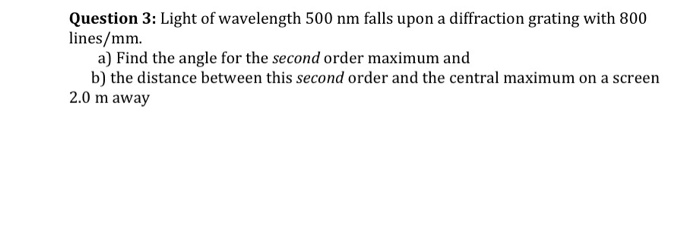Question
A 500 lines per mm diffraction grating is illuminated by light of wavelength 510 nm. What is the angle of each diffraction order?

But I shall mention it anyway:
n ? = d sin(?)

where ? is the angle of emergence, d is distance between slits, ? is the wavelength of the light, n is diffraction order.

all the best!

-EDIT-
alright, since you 'suck' at physics [come on, you can do it if you really think you can], I'll work out some bits for you.

500 lines per mm means 500 000 lines per m
so the distance between each slit is 0.000002 m = 2 x 10^-6 m. always use SI units.

let's do order 1 first. easiest.

your wavelength is 510nm = 5.1 x 10^-7 m

sub in the values and work it out!

bring sin? to one side, so you get sin? = something.
then function sin^-1 to your value for sin?. you will get your ?.

all the best!
there are three diffraction orders. Their angles are: 14.8, 30.7, 49.9

#### Earn Coins

Coins can be redeemed for fabulous gifts.

Similar Homework Help Questions
• ### A 500 lines per mm diffraction grating is illuminated by light of wavelength 560 nm ....

A 500 lines per mm diffraction grating is illuminated by light of wavelength 560 nm . Part A What is the maximum diffraction order seen Part B: What is the angle of each diffraction order starting from zero diffraction order to the maximum visible diffraction order? Enter your answers in degrees in ascending order separated by commas.

• ### A 500 lines per mm diffraction grating is illuminated by light of wavelength 640 nm .

A 500 lines per mm diffraction grating is illuminated by light of wavelength 640 nm . For the steps and strategies involved in solving a similar problem, you may view a Video Tutor Solution.Part AWhat is the maximum diffraction order seen? Express your answer as an integer. Part B What is the angle of each diffraction order starting from zero diffraction order to the maximum visible diffraction order?

• ### A 480 lines/mm diffraction grating is illuminated by light of wavelength 510 nm . How many...

A 480 lines/mm diffraction grating is illuminated by light of wavelength 510 nm . How many bright fringes are seen on a 4.0-m-wide screen located 2.1 m behind the grating?

• ### A 500 line/mm diffraction grating is illuminated by light of wavelength 510 nm. How many bright...

A 500 line/mm diffraction grating is illuminated by light of wavelength 510 nm. How many bright fringes are seen on a 2.0-m-wide screen located 2.0 m behind thegrating?

• ### A diffraction grating with 600 lines/mm is illuminated with light of wavelength 510 nm. A very...

A diffraction grating with 600 lines/mm is illuminated with light of wavelength 510 nm. A very wide viewing screen is 4.2 m behind the grating. Part A What is the distance between the two m = 1 bright fringes? Express your answer with the appropriate units. Δy = SubmitMy AnswersGive Up Part B How many bright fringes can be seen on the screen? N = SubmitMy AnswersGive Up

• ### A 500 line/mm diffraction grating is illuminated by light of wavelength 510nm. how many diffraction orders...

A 500 line/mm diffraction grating is illuminated by light of wavelength 510nm. how many diffraction orders are seen, and what is the angle of each?

• ### A 500 line/mm diffraction grating is illuminated by light of wavelength 510m

A 500 line/mm diffraction grating is illuminated by light of wavelength 510m. How many bright fringes are seen on a 2.0-m-wide screen located behind the grating?

• ### If the scientist uses a diffraction grating with 500 lines per mm and laser with a...

If the scientist uses a diffraction grating with 500 lines per mm and laser with a wavelength of 680 nm, what angle will a line from the grating to the second order bright spot make with the line from the grating to the central bright spot?

• ### Question 3: Light of wavelength 500 nm falls upon a diffraction grating with 800 lines/mm. a)...Question 3: Light of wavelength 500 nm falls upon a diffraction grating with 800 lines/mm. a) Find the angle for the second order maximum and b) the distance between this second order and the central maximum on a screen 2.0 m away

• ### Light with wavelength 405nm incidents on a diffraction grating with 1000 lines per mm. At what...

Light with wavelength 405nm incidents on a diffraction grating with 1000 lines per mm. At what angle is the 1st order diffracted beam found?